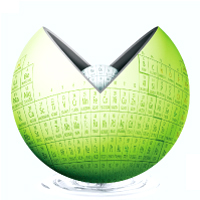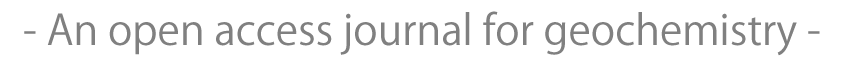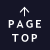JavaScript requeired.Published for geochemistry community from Geochemical Society of Japan.

## Empirical expressions of quartz solubility in H2O, H2O + CO2, and H2O + NaCl fluids

Yasuhiro Shibue
Geochemical Journal, Vol. 30, No. 6, P. 339-354, 1996

#### ABSTRACT

An empirical equation of quartz solubility in H2O at elevated pressures and temperatures (up to 10 kb and 900°C) is obtained based on the published experimental results. The resultant solubility equation is as follows: lnSwater = –1.51914 + 1.99970lnρwater – 2636.78T–1 + 0.00387766T + 0.0198582PT–1, where Swater, Pwater, T, and P stand for quartz solubility (mol/kg H2O), density (g/cm3) of pure water, temperature (Kelvin), and pressure (bar), respectively. Densities of pure water are calculated by the equation of Haar et al. (1984). The solubility equation is then modified for describing the solubility behavior of quartz in H2O + CO2 or H2O + NaCl fluid. For the modification, this study uses the weight fraction of water in the solvent (F), density of the aqueous fluid (ρaq), and an empirical constant (Θ) as the additional terms. The ratio of quartz solubility in aqueous fluid (Saq) to that in pure water (Swater) at the same pressure-temperature condition is then expressed as ln(Saq/Swater) = Θln(ρaqFwater). Densities of H2O + CO2 and H2O + NaCl fluids are computed with the equations of Kerrick and Jacobs (1981) and Anderko and Pitzer (1993a), respectively. The empirical constant for quartz solubility in H2O + CO2 fluid is 1.49063. For obtaining the constant, this study considers the published experimental results done at 1.5 kb to 5 kb, 600° to 700°C, and up to 71 wt% CO2 concentration (mole fraction of CO2 = 0.505). The O value for quartz solubility in H2O + NaCl fluid is 3.24740, which gives the calculated solubilities consistent with the experimental results done at 0.2 kb to 2 kb, 200° to 700°C, and up to 33.6 wt% NaCl concentration.

## All Issues

Current Issue:
Stats:
Impact Factor: 1.060 (2021)
Submission to final decision: 9.6 weeks (2022)